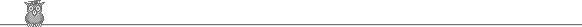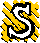### 50th Putnam 1989A1.  Which members of the sequence 101, 10101, 1010101, ... are prime?A2.  Let R be the rectangle 0 ≤ x ≤ a, 0 ≤ y, ≤ b. Evaluate ∫R ef(x, y) dx dy, where f(x, y) = max(b2x2, a2y2).A3.  Prove that all the roots of 11z10 + 10i z9 + 10i z - 11 = 0 have unit modulus.A4.  A player plays the following game. At each turn a fair coin is tossed (probability 1/2 of heads, and all tosses independent), and, depending on the results of the tosses to date, (1) the game ends and the player wins, (2) the game ends and the player loses, or (3) the coin is tossed again. Given an irrational p in the interval (0, 1), can we find a rule such that (A) the player wins with probability p, and (B) the game ends after a finite number of tosses with probability 1?A5.  Show that we can find α > 0 such that, given any point P inside a regular polygon with an odd number of sides which is inscribed in a circle radius 1, we can find two vertices of the polygon whose distance from P differs by less than 1/n - α/n3, where the polygon has 2n + 1 sides.A6.  Let α = 1 + a1x + a2x2 + ... where an = 1 if every block of zeros in the binary expansion of n has even length, 0 otherwise. Prove that, if we calculate in the field of two elements, then α3 + xα + 1 = 0. [For example, calculating in this field, (1 + x)2 = 1 + x + x + x2 = 1 + x2.]B1.  S is a square side 1. R is the subset of points closer to its center than to any side. Find the area of R.B2.  Let S be a non-empty set with a binary operation (written like multiplication) such that: (1) it is associative; (2) ab = ac implies b = c; (3) ba = ca implies b = c; (4) for each element, the set of its powers is finite. Is S necessarily a group?B3.  Let R be the reals and R* the non-negative reals. f: R* → R satisfies the following conditions: (1) it is differentiable and f '(x) = - 3 f(x) + 6 f(2x) for x > 0; (2) |f(x)| ≤ e-√x for x ≥ 0. Define un = ∫0∞ xnf(x) dx for n ≥ 0. Express un in terms of u0, prove that the sequence un3n/n! converges, and show that the limit is 0 iff u0 = 0.B4.  Does there exist an uncountable set of subsets of the positive integers such that any two distinct subsets have finite intersection?B5.  A quadrilateral is inscribed in a circle radius 1. Two opposite sides are parallel. The difference between their lengths is d > 0. The distance from the intersection of the diagonals to the center of the circle is h. Find sup d/h and describe the cases in which it is attained.B6.  f is a continuous real-valued function on the closed interval [0, 1] such that f(1) = 0. A point (a1, a2, ... , an) is chosen at random from the n-dimensional region 0 < x1 < x2 < ... < xn < 1. Define a0 = 0, an+1 = 1. Show that the expected value of ∑0n (ai+1 - ai) f(ai+1) is ∫01 f(x) p(x) dx, where p(x) is a polynomial of degree n which maps the interval [0, 1] into itself (and is independent of f).To avoid possible copyright problems, I have changed the wording, but not the substance of all the problems. The original text of the problems and the official solutions are in American Mathematical Monthly 98 (1991) 321-7.

Putnam home